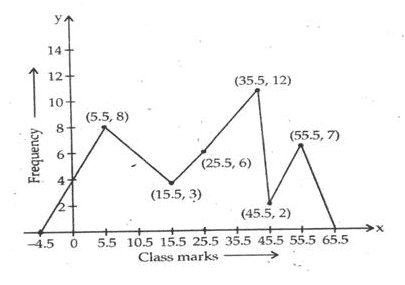# RS Aggarwal Class 9 Solutions Chapter 14 -Statistics Ex 14B (14.2)

## RS Aggarwal Class 9 Chapter 14 -Statistics Ex 14B (14.2) Solutions Free PDF

Q.1: The daily wages of 50 workers in a factory are given below:

 Daily Wages (in rupees) 140-180 180-220 220-260 260-300 300-340 340-380 No. of workers 16 9 12 2 7 4

Construct a histogram to represent the above frequency distribution.

Sol:

Frequency distribution is as below:

 Daily wages ( in Rs.) 140-180 180-220 220-260 260-300 300-340 340-380 No. of workers 16 9 12 2 7 4

In the class intervals, if the upper limit of one class is the lower limit of the next class, it is known as the exclusive method of classification.

Clearly, the given frequency distribution is in the exclusive form.

To draw the required histogram, take the class intervals, i.e. daily wages (in Rs.) along x-axis and frequencies i.e. no. of workers along the y-axis and draw rectangles. So, we get the required histogram.

Since the scale on X-axis starts at 140, a break is indicated near the origin to show that the graph is drawn to scale beginning at 140.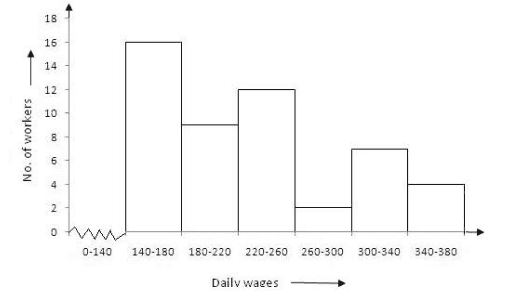Q.2: The following table shows the average daily earnings of 40 general stores in a market, during a certain week:

 Daily earnings (in Rs.) 600-650 650-700 700-750 750-800 800-850 850-900 No. of stores 6 9 2 7 11 5

Draw a histogram to represent the above data.

Sol:

Frequency distribution is as below:

 Daily earnings (in Rs.) 600-650 650-700 700-750 750-800 800-850 850-900 No. of stores 6 9 2 7 11 5

In the class intervals, if the upper limit of one class is the lower limit of the next class, it is known as the exclusive method of classification.

Clearly, the given frequency distribution is the exclusive form.

We take class intervals, i.e. daily earnings (in Rs.) along x-axis and frequencies i.e. the number of stores along y-axis. So, we get the required histogram.

Since the scale on x-axis starts at 600, a kink (break) is indicated near the origin to show that the graph is drawn to scale beginning at 600.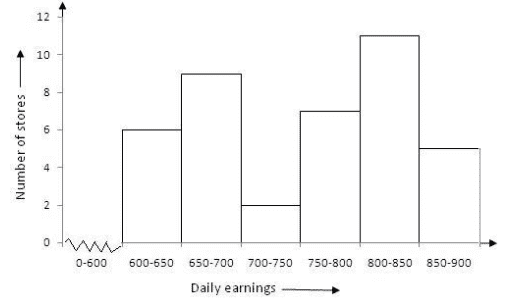Q.3: The height of 75 students in a school is given below:

 Height (in cm) 130-136 136-142 142-148 148-154 154-160 160-166 No. of students 9 12 18 23 10 3

Draw a histogram to represent the above data.

Sol:

The frequency distribution is as below:

 Height (in cm) 130-136 136-142 142-148 148-154 154-160 160-166 No. of students 9 12 18 23 10 3

In the class intervals, if the upper limit of one class is the lower limit of the next class, it is known as the exclusive method of classification.

Clearly, the given frequency distribution is in the exclusive form.

We take class intervals, i.e. height (in cm) along x-axis and frequencies i.e. the number of students along the y-axis. So we get the required histogram.

Since the scale on x-axis starts at 130, a kink (break) is indicated near the origin to show that the graph is drawn to scale beginning at 130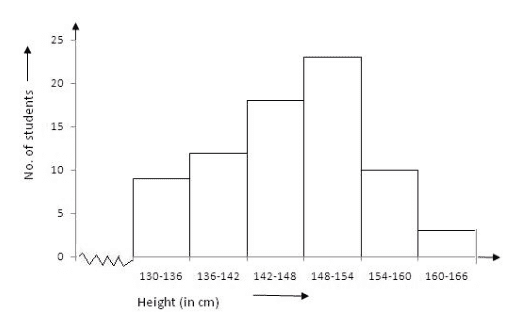Q.4: Draw a histogram for the frequency distribution of the following data.

 Class interval 8-13 13-18 18-23 23-28 28-33 33-38 38-43 Frequency 320 780 160 540 260 100 80

Sol:

The frequency distribution is as below:

 Class interval 8-13 13-18 18-23 23-28 28-33 33-38 38-43 Frequency 320 780 160 540 260 100 80

In the class intervals, if the upper limit of one class is the lower limit of the next class, it is known as the exclusive method of classification.

Clearly, the given frequency distribution is in the exclusive form.

We take class intervals along the x-axis and frequency along the y-axis. So, we get the required histogram.

Since the scale on x-axis starts at 8, a kink (break) is indicated near the origin to show that the graph is drawn to scale beginning at 8.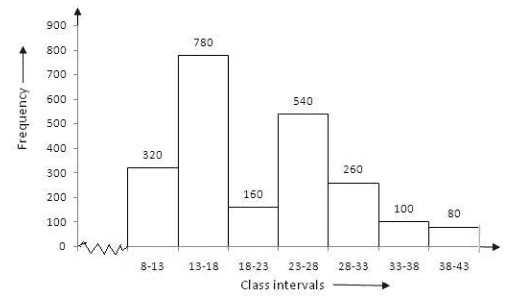Q.5: Construct a histogram for the frequency distribution.

 Class interval 5-12 13-20 21-28 29-36 37-44 45-52 Frequency 6 15 24 18 4 9

Sol:

The histogram is the graphical representation of a frequency distribution in the form of rectangles, such that there is no gap between any two successive rectangles.

Clearly, the given frequency distribution is in inclusive form, that is there is a gap between the upper limit of a class and the lower limit of the next class.

Therefore, we need to convert the given frequency distribution into exclusive form, as shown below:

 Class interval 4.5-12 12.5-20.5 20.5-28.5 28.5-36.5 36.5-44.5 44.5-52.4 Frequency 6 15 24 18 4 9

To draw the required histogram, take class intervals, along x-axis and frequencies along y-axis and draw rectangles. So, we get the required histogram.

Since the scale on x-axis starts at 4.5, a kink (break) is indicated near the origin to show that the graph is drawn to scale beginning at 4.5.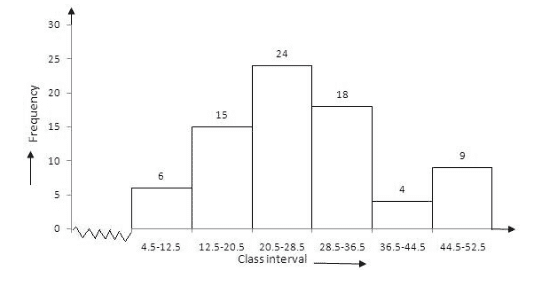Q.6: The following table shows the number of illustrating persons in the age group (10-58 years) in a town:

 Age group (in years) 10-16 17-23 24-30 31-37 38-44 45-51 52-58 No. of illiterate persons 175 325 100 150 250 400 525

Draw a histogram to represent the above data.

Sol:

Given frequency distribution is as below:

 Age group (in years) 10-16 17-23 24-30 31-37 38-44 45-51 52-58 No. of illiterate persons 175 325 100 150 250 400 525

The histogram is the graphical representation of a frequency distribution in the form of rectangles, such that there is no gap between any two successive rectangles.

Clearly, the given frequency distribution is in inclusive form, that is there is a gap between the upper limit of a class and the lower limit of the next class.

Therefore, we need to convert the frequency distribution in exclusive form, as shown below:

 Age group ( in years) 9.5-16.5 16.5-23.5 23.5-30.5 30.5-37.5 37.5-44.4 44.5-51.5 51.5-58.5 No. of illiterate persons 175 325 100 150 250 400 525

To draw the required histogram, take class intervals, that is the age group, along x-axis and frequencies, that is the number of illiterate persons along the y-axis and draw rectangles. So, we get the required histogram.

Since the scale on x-axis starts at 9.5, a kink (break) is indicated near the origin to show that the graph is drawn to scale beginning at 9.5.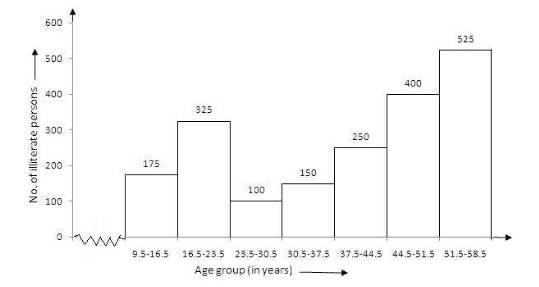Q.7: Draw a histogram to represent the following data.

 Class interval 10-14 14-20 20-32 32-52 52-80 Frequency 5 6 9 25 21

Hint: The adjusted frequencies of the given class intervals are:

(4/4 *5), (4/6 * 6) , (4/12 * 9) , (4/20 *25) , (4/28 * 21) respectively. Note that smallest class size is 4.

Sol:

 Class interval 10-14 14-20 20-32 32-52 52-80 Frequency 5 6 9 25 21

In the above table, class intervals are of unequal size, so we calculate the adjusted frequency by using the following formula:

Adjusted Frequency = Minimumclasssize×itsfrequencyClasssizeofthisclass$\frac{Minimum\; class \;size\times its frequency}{Class\; size \;of \;this \;class}$

Thus, the adjusted frequency table is:

 Class Intervals Frequency Adjusted Frequency 10-14 5 45×5=5$\frac{4}{5}\times 5 =5$ 14-20 6 46×6=4$\frac{4}{6}\times 6 =4$ 20-32 9 412×9=3$\frac{4}{12}\times 9 =3$ 32-52 25 420×25=5$\frac{4}{20}\times 25 =5$ 52-80 21 428×21=3$\frac{4}{28}\times 21 =3$

Now, take class intervals along x-axis and adjusted frequency along y-axis and constant rectangles having their bases as class-size and height as the corresponding adjusted frequencies.

Thus, we obtain the histogram as shown below: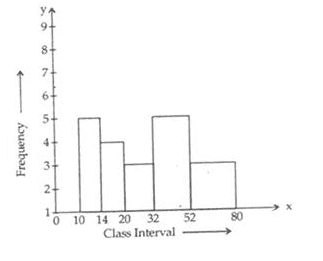Q8: In a study of diabetic patients in a village, the following observations were noted.

 Age in years 10-20 20-30 30-40 40-50 50-60 60-70 No. of patients 2 5 12 19 9 4

Represent the above data by a frequency polygon.

Sol:

 Age in years 10-20 20-30 30-40 40-50 50-60 60-70 No. of patients 2 5 12 19 9 4

In order to draw, frequency polygon, we require class marks. The class mark of a class interval is:

upperlimit+lowerlimit2$\frac{upper\; limit + lower \; limit}{2}$

The frequency distribution table with class marks is given below:

upperlimit+lowerlimit2$\frac{upper\; limit + lower \; limit}{2}$

The frequency distribution table with class marks is given below:

 Class interval Class marks Frequency 0-10 5 0 10-20 15 2 20-30 25 5 30-40 35 12 40-50 45 19 50-60 55 9 60-70 65 4 70-80 75 0

In the above table, we have taken imaginary class intervals 0-10 at beginning and 70-80 at the end, each with frequency zero. Now take class marks along x-axis and the corresponding frequencies along y-axis.

Plot points (5, 0), (15, 2), (25, 5), (35, 12), (45, 19), (55, 9), (65, 4) and (75, 0) and draw line segments.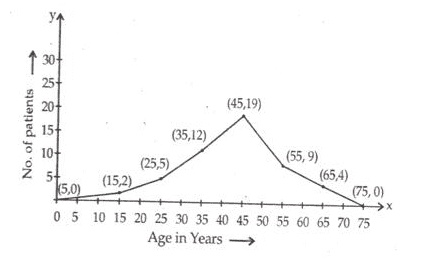Q.9: The ages (in years) of 360 patients treated in a hospital on a particular day are given below.

 Age in years 10-20 20-30 30-40 40-50 50-60 60-70 Number of patients 90 40 60 20 120 30

Draw a histogram and a frequency polygon on the same graph to represent the above data.

Sol:

The given frequency distribution is as below:

 Age in years 10-20 20-30 30-40 40-50 50-60 60-70 Number of patients 90 40 60 20 120 30

Take class intervals i.e. age in years along x-axis and number of patients of width equal to the size of the class intervals and height equal to the corresponding frequencies.

Thus we get the corresponding frequencies.

Thus we get the required histogram.

In order to draw frequency polygon, we take imaginary intervals 0-10 at the beginning and 70-80 at the end each with frequency zero and join the mid-points of the top of the rectangles.

Thus, we obtain a complete frequency polygon, shown below: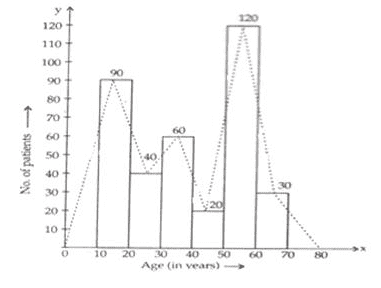Q.10: Draw a histogram and the frequency polygon from the following data.

 Class intervals 20-25 25-30 30-35 35-40 40-45 45-50 Frequency 30 24 52 28 46 10

Sol:

The given frequency distribution is as below:

 Class intervals 20-25 25-30 30-35 35-40 40-45 45-50 Frequency 30 24 52 28 46 10

Take class intervals along x-axis and frequency along the y-axis and draw rectangles of width equal to the size of the class intervals and height equal to the corresponding frequencies. Thus we get required histogram.

Now take imaginary class intervals 15-20 at the beginning and 50-55 at the end, each with frequency zero and joins the midpoint of the top of the rectangle to get the required frequency polygon.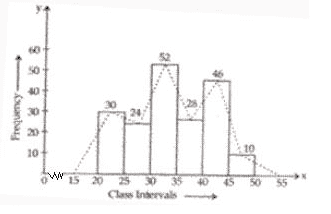Q.11: Draw a histogram for the following data:

 Class Interval 600-640 640-680 680-720 720-760 760-800 800-840 Frequency 18 45 153 288 171 63

Using this histogram, draw the frequency polygon on the same graph.

Sol:

Take class intervals along the y-axis and draw rectangles of width equal to the size of class intervals and height equal to their corresponding frequencies. Thus we get the required histogram.

Take imaginary class intervals 560-600 at the beginning and 840-880 at the end, each with frequency zero.

Now join the mid points of the top of the rectangles to get the required frequency polygon.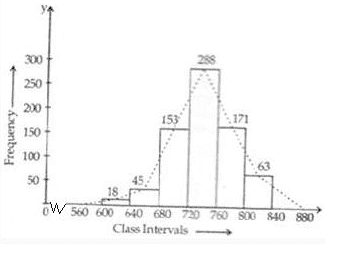Q.12: Draw a frequency polygon for the following frequency distribution:

 Class intervals 1-10 11-20 21-30 31-40 41-50 51-60 Frequency 8 3 6 12 2 7

Sol:

The given frequency distribution table is as below:

 Class intervals 1-10 11-20 21-30 31-40 41-50 51-60 Frequency 8 3 6 12 2 7

This table has inclusive class intervals and so these are to be converted into exclusive intervals (i.e. true class limits).

These are (0.5-10.5), (10.5-20.5),(20.5-30.5),(30.5-40.5),(40.5-50.5), and (50.5-60.5).

In order to draw a frequency polygon, we need to determine the class marks. Class marks of a class interval =upperlimit+lowerlimit2$\frac{upper\; limit + lower \; limit}{2}$

Take imaginary class interval (-9.5 – 0.5) at the beginning and (60.5 – 70.5)  at the end, each with frequency zero. So we have the following table

 Class intervals True class Intervals Class marks Frequency (-9)-0 (-9.5)-0.5 -4.5 0 1-10 0.5-10.5 5.5 8 11-20 10.5-20.5 15.5 3 21-30 20.5-30.5 25.2 6 31-40 30.5-40.5 35.5 12 41-50 40.5-50.5 45.5 2 51-60 50.5-60.5 55.5 7 61-70 60.5-70.5 65.5 0

Now, take class marks along x-axis and their corresponding along y-axis.

Mark the points and join them.

Thus, we obtain a complete frequency polygon as shown below: